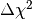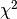# Fitting SEDs to sources¶

## Typical workflow¶

Once the data has been prepared following the description in Data format, and that you have a set of models in the format described in Creating model packages the typical workflow to follow when using the SED fitting tool is:

• Fit a given set of models to the data
• Optionally split the output between well- and badly-fit sources
• Make plots of the SED fits, or of the parameter distribution

Each of these steps can be performed by one ore more functions in the SED Fitting tool, which are described below in detail:

## Note on quantities and units¶

The Python SED fitting tool makes use of the astropy.units package to handle units and unit conversions. Several of the options that need to be specified in the functions described below require Quantity instances. Defining quantities is straightforward:

from astropy import units as u

# Define scalar quantity
q1 = 3. * u.kpc

# Define array quantity using a list
q2 = [1., 2., 3.] * u.arcsec

# Define array quantity using a Numpy array
q3 = np.array([1., 2., 3.]) * u.cm ** 2 / u.g


## Fitting the models to the data¶

In order to fit the data, you will need to make use of the fit() function, which can be imported using:

from sedfitter import fit


At a minimum, you will need to call the function with the following input:

• The file containing the data (see Data format).
• The list of filters and aperture radii for which the data is given (see Common filter names).
• The directory containing the models (see Available Model Packages and Creating model packages).
• The name of the output file
• The range of Av and distances to assume for the fits
• An extinction law, given as a opacities to extinction (in cm^2/g or equivalent) versus wavelength.

The fit() page describes in detail how each of these inputs should be specified, and also lists additional options to further fine-tune the fitting and the output.

The following is a complete example showing how to use the fit() function:

from astropy import units as u
from sedfitter import fit
from sedfitter.extinction import Extinction

# Define path to models
model_dir = '/Volumes/Data/models/models_r06'

extinction = Extinction.from_file('kmh94.par', columns=[0, 3],
wav_unit=u.micron, chi_unit=u.cm**2 / u.g)

# Define filters and apertures
filters = ['2J', '2H', '2K', 'I1', 'I2', 'I3', 'I4']
apertures = [3., 3., 3., 3., 3., 3., 3.] * u.arcsec

# Run the fitting
fit('data_glimpse', filters, apertures, model_dir,
'output.fitinfo',
extinction_law=extinction,
distance_range=[1., 2.] * u.kpc,
av_range=[0., 40.])


Note

in the filter list, you can also specify wavelengths as Astropy Quantity instances. If you do this, the SED wavelength closest to that specified will be used in the fitting.

Note

if you do not specify the columns and units when reading in the extinction, the first two columns are read and are assumed to be in c.g.s.. If you have previously used the Fortran version of the SED fitter, you will need to specify columns=[0, 3] to choose the first and fourth column.

## Plotting SEDs¶

Once you have fit the data, you will likely want to plot the resulting SED fits. To do this, you will need to make use of the plot() function, which can be imported with:

from sedfitter import plot


The plot() requires the output file from the fit() function as well as the name of an output directory. For example, continuing the example above, you can do:

from sedfitter import plot
plot('output.fitinfo', 'plots_seds')


By default, only the best-fit parameter is shown, but this can be changed by using the select_format option, which is described in more detail in Model selection syntax. For example, to write out all the models with avalue per data point (relative to the best fit) of less than 3, you can do:

plot('output.fitinfo', 'plots_seds', select_format=('F', 3))


In addition, there are many options available to customize the format and appearance of the plots. For more information about these options, see the plot() page.

## Plotting parameters¶

Functions are available to make 1- and 2-d parameter plots:

from sedfitter import plot_params_1d, plot_params_2d


As when Plotting SEDs, one needs to specify the output file from the fit() function, the output directory, and the name of the parameters to plot:

from sedfitter import plot_params_1d, plot_params_2d

# Make histograms of the disk mass
plot_params_1d('output.fitinfo', 'MDISK', 'plots_mdisk',
log_x=True)

# Make 2-d plots of the envelope infall rate vs disk mass
plot_params_2d('output.fitinfo', 'MDISK', 'MDOT', 'plots_mdot_mdisk',
log_x=True, log_y=True)


By default, only the best-fit parameter is shown, but this can be changed by using the select_format option, which is described in more detail in Model selection syntax. In addition, there are many options available to customize the format and appearance of the plots. For more information about these options, see the plot_params_1d() and plot_params_2d() pages.

## Splitting well- and badly-fit sources¶

After computing the fits with fit(), it is possible to split the output file on the basis of thevalue for the best-fit. This is done using the filter_output() function which is imported with:

from sedfitter import filter_output


For example, to split the above file into well- and badly-fit sources based on the absoluteof the best-fit, you can do:

filter_output('output.fitinfo', chi=3.)


This will produce files named output_good.fitinfo and output_bad.fitinfo by default (although you can also specify custom names for the output files). It is also possible to split the fits based on thevalue per datapoint using the cpd option. More information about the available options is available in filter_output().

## Extracting the fit and model parameters¶

The output files produced above are in binary format and are not human-readable. To produce ASCII files of the output, you can use the write_parameters() and write_parameter_ranges() functions. The former is used to write out all the parameters of all the models requested, while the latter will only write out the minimum and maximum for each parameter. The functions are imported with:

from sedfitter import write_parameters, write_parameter_ranges


To use these functions, you will need to specify the input binary file, and the output ASCII file name:

from sedfitter import write_parameters, write_parameter_ranges

# Write out all models with a delta chi^2-chi_best^2 per datapoint < 3
write_parameters('output.fitinfo', 'parameters.txt',
select_format=('F', 3.))

# Write out the min/max ranges corresponding to the above file
write_parameter_ranges('output.fitinfo', 'parameter_ranges.txt',
select_format=('F', 3.))


More information about the available options is given in write_parameters() and write_parameter_ranges().